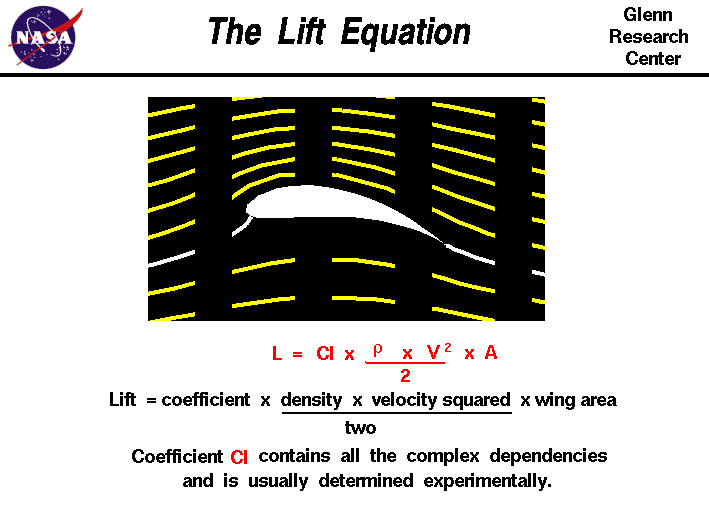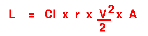+ Text Only Site
+ Non-Flash Version
+ Contact Glenn## Investigating the Lift Equation ActivityIf so instructed by your teacher, print out a worksheet page for these problemsProblems:

1. What do we need to know in order to find the value of L in the equation above?

2. What would we have to do to the equation if we wanted to find the value of Cl instead?

3. Complete the following table and use the results to solve the problems below.

 Variable to solve for: Original equation & work: Final Equation: LClrVA4. Suppose you are flying a 17,160 pound aircraft at 48,000 ft where the air density is 0.0004 slugs/cu. ft. Your current cruising speed is 180 mph and the wing area of the aircraft is 2000 sq. ft. You need to make some altitude changes and will need to know the "Lift Coefficient" of the aircraft in order to do so. What is the Cl for your aircraft?

5. Due to heavy air traffic in the area at 48,000 ft., you are instructed to descend to 30,000 ft. where the air density is 0.00089 slugs/cu. ft. The weight and dimensions of the aircraft have not changed, so you will need to adjust your speed for the new cruising altitude. What should the new V be when you level off at 30,000 ft.?

6. After some time, 500 pounds of fuel have been used and the aircraft now weighs less. Assuming you have not adjusted your speed since you began to cruise at 30,000 ft., what is the air density r for your current altitude?

7. After landing and unloading your cargo, your aircraft is refueled and you take off again for the return trip. The tower directs you to ascend to 25,000 ft. where the air density is 0.00107 slugs/cu. ft. You do so and level off. You are now flying at 80 mph and have the aircraft on autopilot. Out of curiosity, you decide to calculate how much your cargo weighed. Naturally, you'll need to know how much your aircraft weighs now so that it can be compared to the weight with all the cargo. How much does the aircraft weigh (L) now? How much did the cargo weigh?

8. Start the FoilSim program.

Set the following conditions:

Airspeed = 100 mph
Altitude = 0 feet
Angle = 0 degrees
Thickness = 12.5%
Camber = 0
Area = 1.0 sq ft

Angle = 0 degrees
Thickness = 12.5%
Camber = 5.0%

9. Test your calculations by entering the appropriate variable values for each problem. Does FoilSim agree with your calculations? If not, where do they differ? What sources of errors can you find?

Related Pages:
Standards
Worksheet
Lesson Index
Aerodynamics Index+ Inspector General Hotline + Equal Employment Opportunity Data Posted Pursuant to the No Fear Act + Budgets, Strategic Plans and Accountability Reports + Freedom of Information Act + The President's Management Agenda + NASA Privacy Statement, Disclaimer, and Accessibility CertificationEditor: Tom Benson NASA Official: Tom Benson Last Updated: Thu, Jun 12 04:47:15 PM EDT 2014 + Contact Glenn